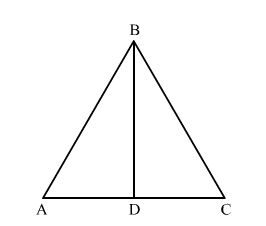# D is any point on the side AC of ΔABC with AB = AC. Show that CD < BD.

Question:

D is any point on the side AC of ΔABC with AB AC. Show that CD BD.

Solution:Given: In ">ΔABCAB = AC

To prove: CD BD

Proof:

In ">ΔABC,

Since, AB = AC       (Given)

So, $\angle A B C=\angle A C B$

In $\Delta A B C$ and $\Delta D B C$,

$\angle A B C>\angle D B C$

$\Rightarrow \angle A C B>\angle D B C \quad[$ From (i) $]$

$\Rightarrow B D>C D \quad$ (Side opposite to greater angle is longer.)

\$\therefore C D